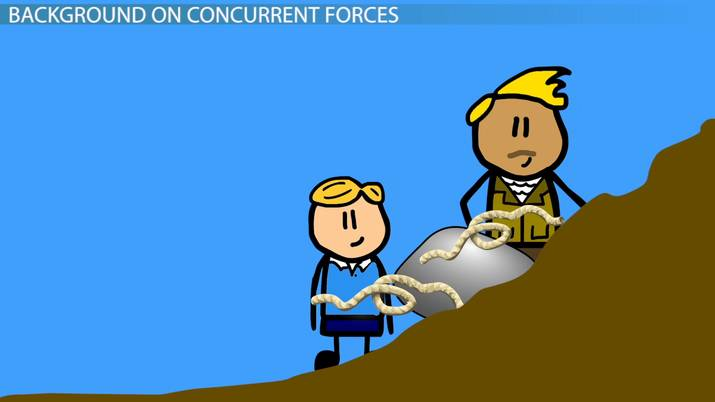Math Courses / Course / Chapter

# Concurrent & Parallel Forces in Physics

Lesson Transcript
Instructor: Betsy Chesnutt

Betsy has a Ph.D. in biomedical engineering from the University of Memphis, M.S. from the University of Virginia, and B.S. from Mississippi State University. She has over 10 years of experience developing STEM curriculum and teaching physics, engineering, and biology.

In physics, force refers to the pull or push that an object experiences when it interacts with another object. Learn about concurrent and parallel forces, understanding how they differ and how to calculate them. Updated: 01/05/2022

## Background on Concurrent Forces

Joe and his father are trying to pull a big rock out of the ground. They know that if they apply a force of 1400 N to the rock, it will move. However, neither Joe nor his father can apply this much force alone.

To try and move the rock, they tie two ropes to the rock and both pull at the same time with equal forces of 1000 N each, but they don't pull in the same direction. Joe pulls his rope due East, while his father pulls due North. Will they be able to move the rock?

To answer this question, we need to know how to calculate the resultant of these two applied forces. The resultant force is the single force that could be applied to an object (in this case, the rock), that would have the same effect as all the applied forces combined.

Because both forces pass through the same point at the center of the rock, we say that these two forces are concurrent. Concurrent forces are forces that all act on the same point. To find the resultant of two concurrent forces, you can't always simply add the two amounts of force together. Why not? Because forces are vectors!

A vector is any quantity with a magnitude and a direction, and the direction is the really critical part here. If Joe and his father were pulling in the same direction, then we could easily find the resultant force by simply adding their two forces together. Similarly, if they were pulling in opposite directions, the resultant force would be zero because we would subtract the force acting to the left (in the negative direction) from the force acting to the right (in the positive direction).

However, in this case, since the forces are perpendicular, we will have to use another technique to find the resultant. One way to find the resultant of two forces is to use the parallelogram rule. To do this, first draw the two force vectors with their tails at the same point, and then draw a parallelogram with those two force vectors as two of the sides of the parallelogram. The resultant will be the diagonal of this parallelogram.

You can use some geometry and trigonometry to find the magnitude and direction of the resultant. To find the magnitude of this resultant force, you can use the Pythagorean theorem. Be careful with this, because it's only valid if there is a right angle in the triangle!

You can find the angle that the resultant force acts using the tangent function, which is equal to the side of the triangle opposite to the angle divided by the side adjacent to the angle.

Let's walk through this. So, the resultant force equals the square root of 1000 N squared plus another 1000 N squared. The resultant force is 1414 N. Since we know the rock will move if a force of 1400 N is applied to it, we now know that Joe and his father will be able to move the rock!An error occurred trying to load this video.

Try refreshing the page, or contact customer support.

Coming up next: Centroid: Definition, Theorem & Formula

### You're on a roll. Keep up the good work!

Replay
Your next lesson will play in 10 seconds
• 0:01 Background on…
• 2:53 Background on Parallel Forces
• 4:42 Lesson Summary
Save Timeline
Autoplay
Autoplay
Speed Speed

## Background on Parallel Forces

Whenever forces are not concurrent, they have the potential to cause an object to rotate. One special case of non-concurrent forces is parallel forces. Parallel forces lie in the same plane and have lines of action that never intersect each other. Two forces that are parallel can either be in the same direction or in opposite directions.

To find the resultant of two parallel forces, you can use the same methods as we used for concurrent forces. However, now you have to also consider where to put the resultant since all the forces are not acting at the same point. Remember that the resultant needs to be equivalent to all the other forces combined.

To unlock this lesson you must be a Study.com Member.

### Register to view this lesson

Are you a student or a teacher?

Back

### Resources created by teachers for teachers

Over 30,000 video lessons & teaching resources‐all in one place.Video lessonsQuizzes & WorksheetsClassroom IntegrationLesson Plans

I would definitely recommend Study.com to my colleagues. It’s like a teacher waved a magic wand and did the work for me. I feel like it’s a lifeline.

Jennifer B.
TeacherCreate an account to start this course today
Used by over 30 million students worldwide